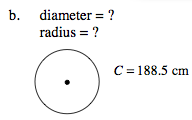### Home > MC2 > Chapter 7 > Lesson 7.1.4 > Problem7-40

7-40.

Find the missing measure(s) of each circle. Homework Help ✎

 a. diameter = ? circumference = ?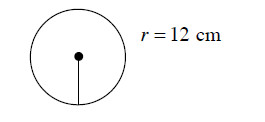b. diameter = ? radius = ?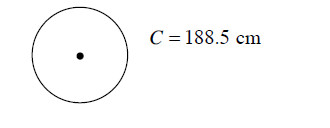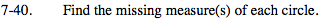Remember:
Circumference = 2 · π · r
Diameter = 2 · r

Use these given formulas to solve for the missing information by substituting in given measurements and solving.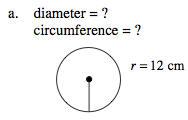Circumference = 24π = 78.4 cm